﻿ 如何評估股票價值

如何評估股票價值當我們買一張股票時，最常碰到的問題是這張股票到底值多少錢？或者用另一角度來問，為何台塑股票80元你願意買？的確！很少人心中有個底，知道想購買的股票實際值多少錢。大部分人買股票的原因是：“覺得”這檔股票會漲，然而這不禁讓我們心中有個疑問，難道沒有一種比較有學理的方法，能夠計算股票的內在價值嗎。

理論模型

P0 = D1/(1+R)1 + D2/(1+R)2 +D3/(1+R)3 +‧‧‧ -----------------------(公式一)

實務演練

觀察最近10年成長率

Excel雖然提供了極佳的計算能力，但是我們還是得預測未來的成長率到底是多少，因為成長率決定股票價值頗巨，所以必須謹慎估算。最好的方式是先了解過去成長情形，再加上最近的研究報告，便比較容易預測未來股息成長情形。最近幾年的股息配發資料網路上應該都不難取得，根據這些資料再做一些運算，就可以得到有用資訊。計算方式是先將每年的配息金額加計配發股票股利後的“實領配息”，再算出每年平均成長率當作未來成長率之參考。有了這些資料，對未來的配息預測是很有幫助的。

考慮股票股利之影響

 欄位標題 儲存格 說明 股票累積 G欄 假設87年買的1股，到了該年度所擁有的股數 還原現金股息 H欄 實質領取股息 = 該年股票累積 * 現金股利 成長率 I 欄 根據還原現金股息，和去年相比之成長率 = (今年股息-去年股息)/去年股息 模擬成長率 J9 模擬股息所根據的成長率之計算參數，意義相當於平均年成長率 模擬股息 J11~J20 計算去年股息以模擬成長率得到之金額 = 去年股息*(1+模擬成長率) 誤差 K11~K20 將模擬股息之當年金額和還原現金股息相減，就是求取這兩者之差距，再平方以得到差距之正值。 = (模擬股息-還原現金股息)2 總誤差 K9 K11~K20之總和，目的是希望得到一個誤差最小的模擬成長率

求出配息平均成長率

87年 = 0.7
88年 = 0.7*(1+36%) = 0.96
89年 = 0.7*(1+36%)*(1+36%) = 1.30
90年 = 0.7*(1+36%)*(1+36%)*(1+36%) = 1.78
.....

1) 先隨便猜一個模擬成長率(J9)，例如20%好了，這時總誤差(儲存格K9)算出來的結果是117，而且模擬股息(圖一粉紅色曲線)看起來和實質股息會有些差異。

2) 試著上下改變儲存格J9的數值，看那一個數字會得到最小的總誤差(K9)，那個成長率就是最接近10年的平均成長率。

▼圖(一)台塑公司最近10年的股利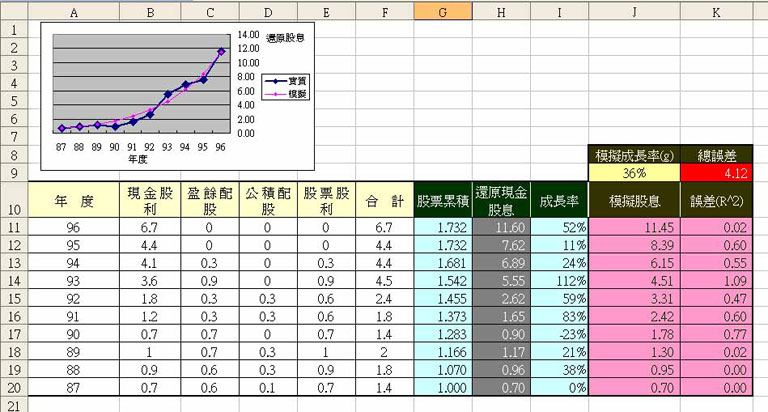利用Excel規劃求解工具

1) 下拉選單=>工具=>規劃求解，會出現如下圖視窗。若工具裡找不到『規劃求解』選項，必須到工具=>增益集裡將『規劃求解』選項打勾才行。
2) 『設定目標儲存格』==>\$K\$9，『等於』==>“最小值”，『變數儲存格』==>\$J\$9
3) 按求解後，如出現“規劃求解找到一解‧‧‧”，選『保存運算結果』，再按『確定』即可

▼圖(二)規劃求解參數設定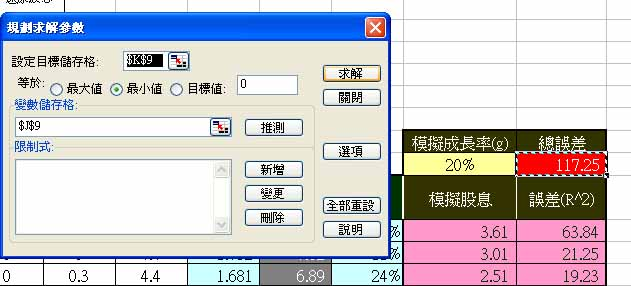計算股票價值

P0 = D1/(1+R)1 + D2/(1+R)2 +D3/(1+R)3 +‧‧‧

▼圖(三)台塑股票價值及成長率預測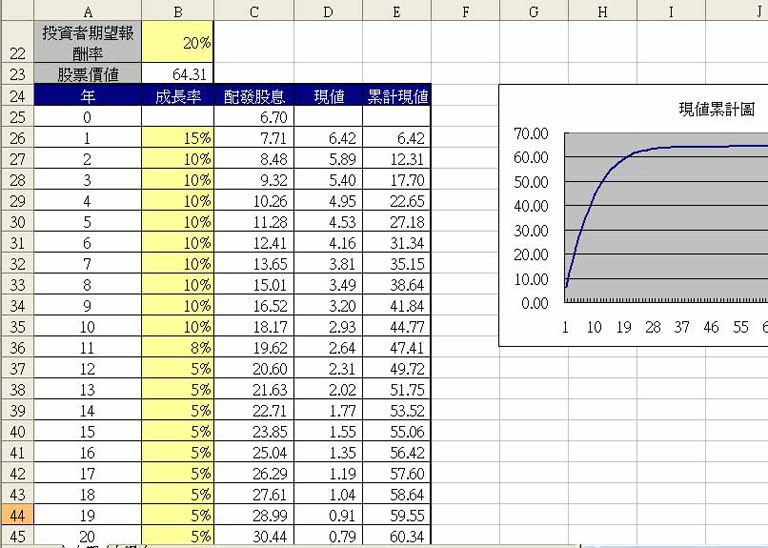網路試算

股票價值試算表

(必填)

% (必填)
預測未來成長率(第1段必填，2~5段選填)

附註一：投資學上的分析方法

配息零成長評價公式

P0 = D/(1+R)1 + D/(1+R)2+ D/(1+R)3+‧‧‧
=D*(1/(1+R)1 + 1/(1+R)2+ 1/(1+R)3+‧‧‧)
=D/R ---------------(公式二)

 報酬率(R) 投資者願意購買價 10% 30 15% 20 20% 15 30% 10

用Excel來計算

P0 = D/R = 3/20% = 15

獲利成長評價公式

P0 = D1/(1+R)1 + D2/(1+R)2 +D3/(1+R)3 +‧‧‧
= D0(1+g)1/(1+R)1 + D0(1+g)2/(1+R)2 + D0(1+g)3/(1+R)3+‧‧‧

P0 = D0*(1+g)/(R-g) = D1/(R-g) ---------------(公式三)
=>

附註二：網站下載配息表

1) 先到奇摩股市，輸入股票號碼或名稱
2) 應該會顯示該股之當日行情，在『個股資料』欄裡點選“基本”，如圖上箭頭所式。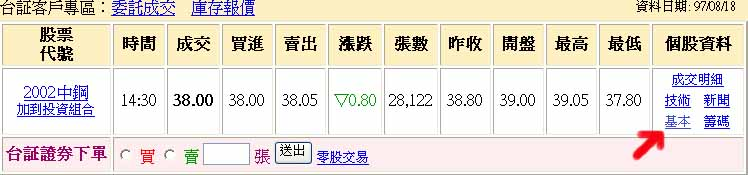3) 在公司資料裡再選擇“股利政策”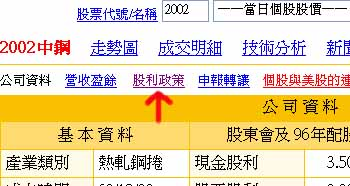4)就可以看到最近10年的配股配息的資料了。裡面欄位和Excel應該是一樣，可以直接從網上複製到Excel的工作表A11~F20的範圍。

﻿
﻿

■ 本網站內容儘可能精確完整，但不保證無誤。若做為投資依據，風險請自行斟酌 ，本網站不負賠償之責任。
■ 網站所有資料均為版權所有，非經書面允許請勿轉載或使用。